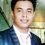# Try this problem!!!!

Suppose f is a function such that $f:\mathbb{Z} ^+\to \mathbb{Z} ^+$ satisfying

$f(1)=1, f(2n)=f(n)$ and $f(2n+1)=f(2n)+1$,

for all positive integers $n$.

Find the maximum of $f(n)$ when $1 \leq n \leq 2013$.Note by Mharfe Micaroz
6 years, 9 months ago

This discussion board is a place to discuss our Daily Challenges and the math and science related to those challenges. Explanations are more than just a solution — they should explain the steps and thinking strategies that you used to obtain the solution. Comments should further the discussion of math and science.

When posting on Brilliant:

• Use the emojis to react to an explanation, whether you're congratulating a job well done , or just really confused .
• Ask specific questions about the challenge or the steps in somebody's explanation. Well-posed questions can add a lot to the discussion, but posting "I don't understand!" doesn't help anyone.
• Try to contribute something new to the discussion, whether it is an extension, generalization or other idea related to the challenge.

MarkdownAppears as
*italics* or _italics_ italics
**bold** or __bold__ bold
- bulleted- list
• bulleted
• list
1. numbered2. list
1. numbered
2. list
Note: you must add a full line of space before and after lists for them to show up correctly
paragraph 1paragraph 2

paragraph 1

paragraph 2

[example link](https://brilliant.org)example link
> This is a quote
This is a quote
    # I indented these lines
# 4 spaces, and now they show
# up as a code block.

print "hello world"
# I indented these lines
# 4 spaces, and now they show
# up as a code block.

print "hello world"
MathAppears as
Remember to wrap math in $$ ... $$ or $ ... $ to ensure proper formatting.
2 \times 3 $2 \times 3$
2^{34} $2^{34}$
a_{i-1} $a_{i-1}$
\frac{2}{3} $\frac{2}{3}$
\sqrt{2} $\sqrt{2}$
\sum_{i=1}^3 $\sum_{i=1}^3$
\sin \theta $\sin \theta$
\boxed{123} $\boxed{123}$

Sort by:

$f(n)$ is the number of $1$s in the binary expansion of $n$. Since $2^{11} = 2048$, the smallest integer $n$ with $f(n) = 11$ is $2047$. Since $f(1983) = 10$, the maximum of $f(n)$ over $1 \le n \le 2013$ is $10$.

- 6 years, 9 months ago

This function doubtlessly suffices the condition, & the solution is beautiful. But is this definition of $f(n)$ unique? Why?

- 6 years, 9 months ago

Each number can be constructed, according to its binary expansion, by applying these rules.

We start with f(1), or "1" in binary. The f(2n) equation will tell us what f provides when we append 0 to our input, that is, f(10), and f(2n+1) tells us what happens when we append a 1.

e.g. f(110101) is computed by f(1) --> f(11) --> f(110) --> f(1101) --> f(11010) --> f(110101).

(all numbers in binary)

- 6 years, 9 months ago

Prove it by (strong) induction on $n$.

- 6 years, 9 months ago

- 6 years, 9 months ago

Beat me to it... although it has been some time since it was posted.

But, yes, although it would have sufficed simply to use $2^{10}-1$ (since there cannot be any more $1$s, as this number would then be $2^{11}-1$).

The argument for the sum of the digits of the binary expansion can be seen by simple construction (this is just a quick, non-rigorous sketch) of a two-case inductive proof:

Allow the number $n$ to be of the form $n=a_k2^{k-1}+a_{k-1}2^{k-2}+\cdots+a_1$, where $a_i\in\{0,1\}$ and $a_k=1$.

Case 1

Then, if this number is odd, we must have $a_1=1$, hence, we have $f(2m+1)=f(2m)+1$ for some $m\in\mathbb{Z}$. So, now our number becomes: $2m+1=a_k2^{k-1}+a_{k-1}2^{k-2}+\cdots+a_22^{1}+1$ $m=a_k2^{k-2}+a_{k-1}2^{k-3}+\cdots+a_2$ This adds unity to our count, removes the last digit, and continues the process until the only value left of $m$ is one.

Case 2

Say that $n$ is even, then we must have $a_1=0$. Clearly, then, we have: $f(2m)=f(m)$, which, after dividing, gives us a new number with the last digit removed and adds nothing to the count (as the last digit is 0 in base 2).

The same process applies.

Hence, we are done (note that this proof can easily be extended for uniqueness of definition as Paramjit S.'s request).

Hopefully this makes the case a little more clear for those in doubt.

- 6 years, 9 months ago

a very good thing indeed.good work Mark H.

- 6 years, 9 months ago

Hi there! Seeing that I am having trouble with starting a discussion, I decided to just post it as a comment instead.

While preparing for the South African National Olympiad, the following problem featured in my training material:

"An 11 sided polygon has its vertices on a circle. How many triangles can be formed using three vertices of the polygon but sharing no side with a side of the polygon?"

Can somebody please provide a solution?

Thanks!

- 6 years, 9 months ago

1009!

- 6 years, 9 months ago

Sorry, it's not 1009 factorial. :) Lol Careful with the !

- 6 years, 9 months ago

Is it 11?

- 6 years, 9 months ago